International
Tables for
Crystallography
Volume C
Mathematical, physical and chemical tables
Edited by E. Prince

International Tables for Crystallography (2006). Vol. C, ch. 6.3, pp. 607-608

## Section 6.3.3.5. Empirical methods

E. N. Maslena

aCrystallography Centre, The University of Western Australia, Nedlands, Western Australia 6009, Australia

#### 6.3.3.5. Empirical methods

| top | pdf |

Some crystals do not have regular faces, or cannot be measured because these are obscured by the crystal mounting. If corrections based on measurements of the crystal shape are not feasible, absorption measurements may be estimated, either from the intensities of the same reflection at different azimuthal angles ψ (see Subsection 6.3.3.6), or from measurements of equivalent reflections, by empirical methods.

There are variants of the method related to differences in experimental technique. The principles may be illustrated by reference to the procedure for a four-circle diffractometer (Flack, 1977).

Intensities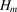are measurements for a reflection S at the angular positions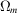,,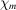,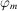. Corrected intensities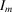are to be derived from the measurements by means of a correction factor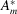such that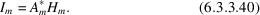It is assumed that the correction can be written in the form of a rapidly converging Fourier series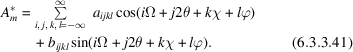The form of the geometrical terms may be simplified by taking advantage of the symmetry of the four-circle diffractometer. If it is assumed that diffraction is invariant to reversal of the incident and diffracted beams, the settings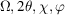;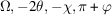;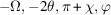;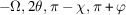;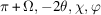;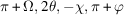;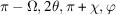;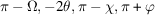are equivalent. In shorthand notation, the series (6.3.3.41)reduces to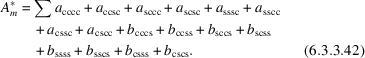The range of indices for some terms may be restricted by noting other symmetries in the diffraction experiment. Thus, equation (6.3.3.40)will define the absorption correction for measurements of the incident-beam intensity, with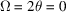. Since with this geometry the correction will be invariant to rotation about the χ axis, the coefficients for the function involving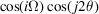must vanish if the χ index, k, is non-zero. By similar reasoning with theaxis along the incident beam, one may deduce that coefficients for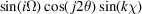will vanish unless l = 0.

Because for a given reflection all measurements are made at the same Bragg angle, thedependence of the correction cannot be determined by empirical methods. This factor in A is obtained from the absorption correction for a spherical crystal of equivalent radius.

Since an empirical absorption correction is defined only to within a scale factor, the scale must be specified by applying a constraint such that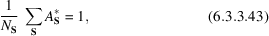where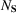is the number of independent reflections. Equation (6.3.3.42)may be expressed in the shorthand notation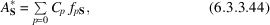where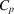is the coefficient in a term such as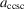or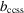and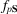is the corresponding geometrical function. Labelling the constant geometrical term with a value of unity asand rearranging leads to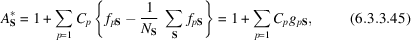which defines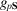.

Equation (6.3.3.40)is now expressed as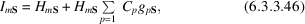in which the coefficientsare to be chosen so that the values of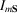for each S are as near equal as possible. Since the values within each set will not be exactly equal, we rewrite (6.3.3.46)as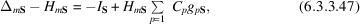in which the mean intensity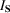and theare chosen to minimize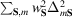, where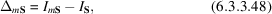and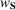is the weight for that reflection.

If the equation to be solved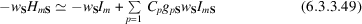is written in the shorthand form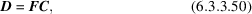in which D corresponds to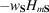, theandcorrespond to C, with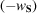and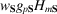corresponding to F, the solution to (6.3.3.50)can be determined from the normal equations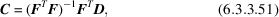where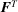is the transpose of F. This procedure suffers from the disadvantages of requiring a matrix inversion whenever the set of trial functions (i.e. those multiplied by the coefficients) is modified. The tedious inversion of the normal equations, described by (6.3.3.51), may be replaced by a simple inversion via the Gram–Schmidt orthogonalizing process, i.e. by calculating a matrix W with mutually orthogonal columnssuch that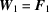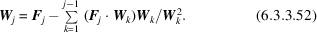The minimizing of (DFC)2 is replaced by minimizing (DWA)2. Differentiating with respect to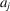yields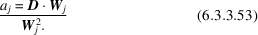If equation (6.3.3.52)is written as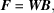where the upper triangular matrix B is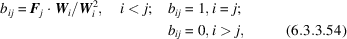the vector determining the coefficients is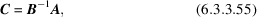in which the inversion of B is straightforward.

In difficult cases, with data affected by errors in addition to absorption, the method described may give physically unreasonable absorption corrections for some reflections. In such cases, it may help to impose the approximate constraints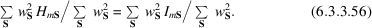If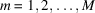, this reduces to the M constraint equations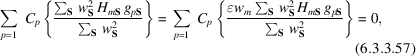where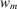is the square root of the weight for the weighted mean of the equivalent reflections, defined as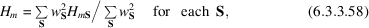and the multipliercontrols the strength with which the additional constraints are enforced. With the additional constraint equations, the sum of squares to be minimized, corresponding to (6.3.3.48), becomes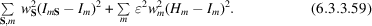A closely related procedure expressing the absorption corrections as Fourier series in polar angles for the incident and diffracted beams is described by Katayama, Sakabe & Sakabe (1972). A similar method minimizing the difference between observed and calculated structure factors is described by Walker & Stuart (1983). Other experimental techniques for measuring data for empirical absorption corrections that could be analysed by the Fourier-series method are described by Kopfmann & Huber (1968), North, Phillips & Mathews (1968), Flack (1974), Stuart & Walker (1979), Lee & Ruble (1977a,b), Schwager, Bartels & Huber (1973), and Santoro & Wlodawer (1980).

### References

Flack, H. D. (1974). Automatic absorption correction using intensity measurements from azimuthal scans. Acta Cryst. A30, 569–573.Google Scholar
Flack, H. D. (1977). An empirical absorption–extinction correction technique. Acta Cryst. A33, 890–898Google Scholar
Katayama, C., Sakabe, N. & Sakabe, K. (1972). A statistical evaluation of absorption. Acta Cryst. A28, 293–295.Google Scholar
Kopfmann, G. & Huber, R. (1968). A method of absorption correction for X-ray intensity measurements. Acta Cryst. A24, 348–351.Google Scholar
Lee, B. & Ruble, J. R. (1977a). A semi-empirical absorption-correction technique for symmetric crystals in single-crystal X-ray crystallography. I. Acta Cryst. A33, 629–637.Google Scholar
Lee, B. & Ruble, J. R. (1977b). A semi-empirical absorption-correction technique for symmetric crystals in single-crystal X-ray crystallography. II. Acta Cryst. A33, 637–641.Google Scholar
North, A. C. T., Phillips, D. C. & Mathews, F. S. (1968). A semi-empirical method of absorption correction. Acta Cryst. A24, 351–359.Google Scholar
Santoro, A. & Wlodawer, A. (1980). Absorption corrections for Weissenberg diffractometers. Acta Cryst. A36, 442–450.Google Scholar
Schwager, P., Bartels, K. & Huber, R. (1973). A simple empirical absorption-correction method for X-ray intensity data films. Acta Cryst. A29, 291–295.Google Scholar
Stuart, D. & Walker, N. (1979). An empirical method for correcting rotation-camera data for absorption and decay effects. Acta Cryst. A35, 925–933.Google Scholar
Walker, N. & Stuart, D. (1983). An empirical method for correcting diffractometer data for absorption effects. Acta Cryst. A39, 158–166.Google Scholar